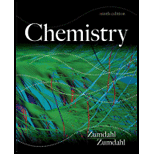# At a given temperature, K = 1.3 × 10 −2 for the reaction N 2 ( g ) + 3 H 2 ( g ) ⇌ 2 NH 3 ( g ) Calculate values of K for the following reactions at this temperature. a .· 1 2 N 2 ( g ) + 3 2 H 2 ( g ) ⇌ NH 3 ( g ) b . 2 N H 3 ( g ) ⇌ N 2 ( g ) + 3 H ( g ) c . NH 3 ( g ) ⇌ 1 2 N 2 ( g ) + 3 2 H 2 ( g ) d. 2N 2 ( g ) + 6 H 2 ( g ) ⇌ 4 NH 3 ( g )### Chemistry

9th Edition
Steven S. Zumdahl
Publisher: Cengage Learning
ISBN: 9781133611097### Chemistry

9th Edition
Steven S. Zumdahl
Publisher: Cengage Learning
ISBN: 9781133611097

#### Solutions

Chapter
Section
Chapter 13, Problem 23E
Textbook Problem

## Expert Solution

### Want to see the full answer?

Check out a sample textbook solution.See solution

### Want to see this answer and more?

Experts are waiting 24/7 to provide step-by-step solutions in as fast as 30 minutes!*

See Solution

*Response times vary by subject and question complexity. Median response time is 34 minutes and may be longer for new subjects.